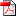#### Seminar 10: Excited states (suggested literature)

Three papers that prove quite comprehensive introduction to TD-DFTSeminar 10: Excited states (suggested literature) [ 4,695 kb ]

#### Introduction to practical quantum chemistry: Seminar 7

Part 7 and 8: Vibrational analysis and transition state searchIntroduction to practical quantum chemistry: Seminar 7 [ 851 kb ]

#### Introduction to practical quantum chemistry: Seminar 6

Part 6: Density Functional Theory (DFT)Introduction to practical quantum chemistry: Seminar 6 [ 4,897 kb ]

#### Introduction to practical quantum chemistry: Seminar 5

Part 5: post-HF methods (CI, CC, MP2), first order propertiesIntroduction to practical quantum chemistry: Seminar 5 [ 937 kb ]

#### Introduction to practical quantum chemistry: Seminar 4

Part 4: post-HF methods and equilibrium distributionsIntroduction to practical quantum chemistry: Seminar 4 [ 627 kb ]

#### Introduction to practical quantum chemistry: Seminar 3

Part 3: Hartree-Fock method and geometry optimisationsIntroduction to practical quantum chemistry: Seminar 3 [ 387 kb ]

#### Introduction to practical quantum chemistry: Seminar 2

Part 2: Hartree-Fock method and basis setsIntroduction to practical quantum chemistry: Seminar 2 [ 394 kb ]